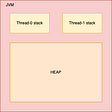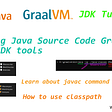# Description

Determine whether an integer is a palindrome. An integer is a palindrome when it reads the same backward as forward.

Example 1:

`Input: 121Output: true`

Example 2:

`Input: -121Output: falseExplanation: From left to right, it reads -121. From right to left, it becomes 121-. Therefore it is not a palindrome.`

Example 3:

`Input: 10Output: falseExplanation: Reads 01 from right to left. Therefore it is not a palindrome.`

Coud you solve it without converting the integer to a string?

# Solution

At first, I answered with the following codes. ( It is the same method as I used in solving the 7. Reverse Integer. related article: https://medium.com/@fukubaka0825/go-leetcode-7-reverse-integer-eaba9692c9a )

`/* Approach 1: Using simple push and pop(my first answer) *//* Time complexity : O(log10(n)) Space complexity: O(1) */func isPalindrome(x int) bool {    if x < 0 {        return false    }        var rev int     dividedx := x        for dividedx != 0 {        //pop        pop := dividedx % 10        dividedx /= 10        //push        rev = rev * 10 + pop    }        return x == rev}`

It was accepted but I thought it is not good enough when I checked the model answer.

So, I revised my answer to use enough validation and reduce loop counts.

`/* Approach 2: Revert half of the number *//* Time complexity : O(log10(n)) Space complexity: O(1) */func isPalindrome(x int) bool {    if x < 0 || (x % 10 == 0 && x != 0) {        return false    }    var rev int    for x > rev {        //pop and push        rev = rev * 10 + x % 10        x /= 10    }    // Considering about both odd and even cases.    return x == rev || x  == rev / 10}`

--

--

--

Site Reliablity Engineer in Tokyo. SRE/devops/Go/AWS/Terraform/Kubernetes/Docker

Love podcasts or audiobooks? Learn on the go with our new app.## TAKASHI NARIKAWA

Site Reliablity Engineer in Tokyo. SRE/devops/Go/AWS/Terraform/Kubernetes/Docker

## Java Microservice Layer Architecture## Concurrency in Java: Executor Service (Part 2)## Compiling and running Java Source code using GraalVM and Oracle Java Development Kit